# Maths - Category Theory - Limits and Colimits

### Limits and Colimits

A limit is a way to take a diagram and encapsulate its properties into one object as simply as possible.

 This is a generalisation that includes: initial/terminal objects (co)products (co)equalizers pullbacks/pushout.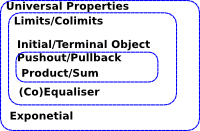## Limits

These generate a construction (c) which depends on the diagram such that:

• All triangles commute.
• Any other object c' can be factored through c without loosing information (there is a unique arrow to make the cones commute).

The first condition makes 'c' general enough to capture the essence of the construction.

The second condition makes sure that it is only just general enough (no junk).

Universal Construction Universal Cones over Diagram:

#### terminal

This is a cone over an empty diagram.

Therefore the diagram puts no constraints on c. It is the most general object and so any other object can map to it.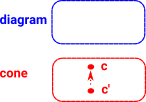#### product

objects 'a' and 'b' are independent of each other so c needs to be general enough to contain the information in both.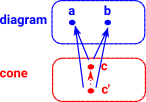#### arrow

Not a necessarily universal construction but I've included it here for completeness. Here there is an arrow between 'a' and 'b' so they are no longer independent.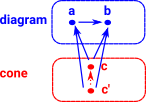#### equaliser

'c' is a subobject of 'a' on which 'f' and 'g' agree. Monics are equalisers in set or any topos.

injective function goes from 'c' to potentially larger objects 'a' and 'b'.

In set an injective function defines a subset relationship. In category theory this can be generalised to a monomorphism (monic).if h•f=h•g then f=g (h on left cancels out if monic)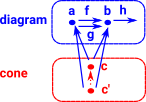#### pullback## Colimits

universal cone over diagram:

#### Initial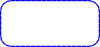empty diagram

#### Sum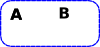#### pushout#### coequaliser

In set an surjective function defines an equivalence relationship. In category theory this can be generalised to a epimorphism (epi).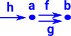if f•h=g•h then f=g (h on right cancels out if epi)

Shows which elements of 'a' are equal to other elements of 'a'. (Can be represented by some subset of a×a).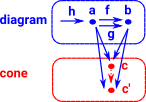## Limits and CoLimits in Set

Just to give some intuition here are some diagrams showing the internal set structure.

universal cones over diagram:

#### terminal

not sure how to show this

#### product

objects 'a' and 'b' are independent of each other so c needs to be general enough to contain the information in both.#### arrow injective

In this example we cant make the triangle commute with a 3 element set in cone.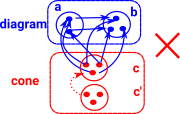#### arrow surjective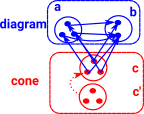#### equaliser

'c' is the subset of 'a' on which 'f' and 'g' agree.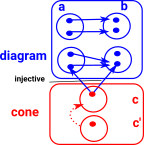#### co-equaliser

Surjective function merges back those elements that don't commute.#### pullback## Relationship to Type Theory

Category theory is deeply related to type theory. We can therefore see these patterns in the constructors and deconstructors(eliminators) of types.

So can we define a type from a diagram? C represents the type we are trying to construct from a diagram.